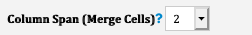# Sum various columns in a subtable

Hi there,

I would like to sum more than 1 column in a Subtable.

Let

Let’s say I colums C with quantities and also column D have some quantities.
I already know how to sum quantities from column C, but I also want to calculate quantities of column D.

Screenshot

Hi,

Please create a numeric field in D24 and apply it with formulas D20.
Remember to apply for the formulas recalculation once the formulas added.

Hi Angie,

Maybe I am doing something wrong.

When I choose the field I want to make the SUM of the above cells, I can’t insert a formula on the Field Settings.

Please check the 2 images bellow:

Image shows the D27 cell I want to make the SUM

Image show there is no Field Settings because there is no filed setected.

Thank you.

Hi,

You’ll need to type in the field name to create the numeric field first. Then, apply the formulas.

Hi Angie,

If I do that, the total will appear on the E31 cell, corretc?

I would like it to appear on the D31 cell.

Thank you.

Hi,

Currently, you’ll need to have a field there first in order to apply formulas.

A workaround would be as below:

Step 1. Span (merge cells) your “#Pallets/Master Box” field from D27 to E27.Step 2. Create a new numeric field in D27 and apply for the formulas
Then, the result would be in E31

Step 3 Tune the column width of the D column so that you won’t see the field title

Hi Angie!

Understood. I will use the workaround.

Thank you.